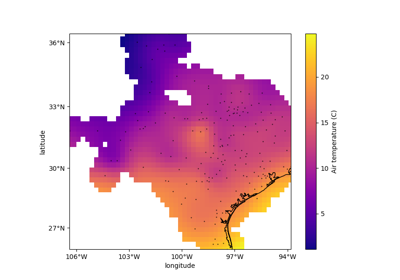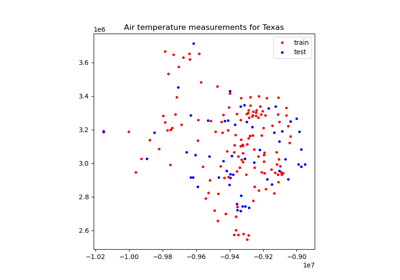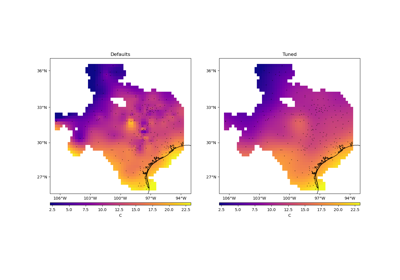# verde.cross_val_score¶

verde.cross_val_score(estimator, coordinates, data, weights=None, cv=None, client=None, delayed=False, scoring=None)[source]

Score an estimator/gridder using cross-validation.

Similar to `sklearn.model_selection.cross_val_score` but modified to accept spatial multi-component data with weights.

By default, will use `sklearn.model_selection.KFold` with `n_splits=5` and `random_state=0` to split the dataset. Any other cross-validation class from scikit-learn or Verde can be passed in through the cv argument.

The score is calculated using the estimator/gridder’s `.score` method by default. Alternatively, use the scoring parameter to specify a different scoring function (e.g., mean square error, mean absolute error, etc).

Can optionally run in parallel using `dask`. To do this, use `delayed=True` to dispatch computations with `dask.delayed` instead of running them. The returned scores will be “lazy” objects instead of the actual scores. To trigger the computation (which Dask will run in parallel) call the .compute() method of each score or `dask.compute` with the entire list of scores.

Warning

The `client` parameter is deprecated and will be removed in Verde v2.0.0. Use `delayed` instead.

Parameters
• estimator (verde gridder) – Any verde gridder class that has the `fit` and `score` methods.

• coordinates (tuple of arrays) – Arrays with the coordinates of each data point. Should be in the following order: (easting, northing, vertical, …).

• data (array or tuple of arrays) – the data values of each data point. If the data has more than one component, data must be a tuple of arrays (one for each component).

• weights (none or array or tuple of arrays) – if not none, then the weights assigned to each data point. If more than one data component is provided, you must provide a weights array for each data component (if not none).

• cv (None or cross-validation generator) – Any scikit-learn or Verde cross-validation generator. Defaults to `sklearn.model_selection.KFold`.

• client (None or dask.distributed.Client) – DEPRECATED: This option is deprecated and will be removed in Verde v2.0.0. If None, then computations are run serially. Otherwise, should be a dask `Client` object. It will be used to dispatch computations to the dask cluster.

• delayed (bool) – If True, will use `dask.delayed` to dispatch computations without actually executing them. The returned scores will be a list of delayed objects. Call .compute() on each score or `dask.compute` on the entire list to trigger the actual computations.

• scoring (None, str, or callable) – A scoring function (or name of a function) known to scikit-learn. See the description of scoring in `sklearn.model_selection.cross_val_score` for details. If None, will fall back to the estimator’s `.score` method.

Returns

scores (array) – Array of scores for each split of the cross-validation generator. If delayed, will be a list of Dask delayed objects (see the delayed option). If client is not None, then the scores will be futures.

`train_test_split`

Split a dataset into a training and a testing set.

`BlockShuffleSplit`

Random permutation of spatial blocks cross-validator.

Examples

As an example, we can score `verde.Trend` on data that actually follows a linear trend.

```>>> from verde import grid_coordinates, Trend
>>> coords = grid_coordinates((0, 10, -10, -5), spacing=0.1)
>>> data = 10 - coords + 0.5*coords
>>> model = Trend(degree=1)
```

In this case, the model should perfectly predict the data and R² scores should be equal to 1.

```>>> scores = cross_val_score(model, coords, data)
>>> print(', '.join(['{:.2f}'.format(score) for score in scores]))
1.00, 1.00, 1.00, 1.00, 1.00
```

There are 5 scores because the default cross-validator is `sklearn.model_selection.KFold` with `n_splits=5`.

To calculate the score with a different metric, use the scoring argument:

```>>> scores = cross_val_score(
...     model, coords, data, scoring="neg_mean_squared_error",
... )
>>> print(', '.join(['{:.2f}'.format(-score) for score in scores]))
0.00, 0.00, 0.00, 0.00, 0.00
```

In this case, we calculated the (negative) mean squared error (MSE) which measures the distance between test data and predictions. This way, 0 is the best possible value meaning that the data and prediction are the same. The “neg” part indicates that this is the negative mean square error. This is required because scikit-learn assumes that higher scores are always treated as better (which is the opposite for MSE). For display, we take the negative of the score to get the actual MSE.

We can use different cross-validators by assigning them to the `cv` argument:

```>>> from sklearn.model_selection import ShuffleSplit
>>> # Set the random state to get reproducible results
>>> cross_validator = ShuffleSplit(n_splits=3, random_state=0)
>>> scores = cross_val_score(model, coords, data, cv=cross_validator)
>>> print(', '.join(['{:.2f}'.format(score) for score in scores]))
1.00, 1.00, 1.00
```

Often, spatial data are autocorrelated (points that are close together are more likely to have similar values), which can cause cross-validation with random splits to overestimate the prediction accuracy [Roberts_etal2017]. To account for the autocorrelation, we can split the data in blocks rather than randomly with `verde.BlockShuffleSplit`:

```>>> from verde import BlockShuffleSplit
>>> # spacing controls the size of the spatial blocks
>>> cross_validator = BlockShuffleSplit(
...     spacing=2, n_splits=3, random_state=0
... )
>>> scores = cross_val_score(model, coords, data, cv=cross_validator)
>>> print(', '.join(['{:.2f}'.format(score) for score in scores]))
1.00, 1.00, 1.00
```

We didn’t see a difference here since our model and data are perfect. See Evaluating Performance for an example with real data.

If using many splits, we can speed up computations by running them in parallel with Dask:

```>>> cross_validator = ShuffleSplit(n_splits=10, random_state=0)
>>> scores_delayed = cross_val_score(
...     model, coords, data, cv=cross_validator, delayed=True
... )
>>> # The scores are delayed objects.
>>> # To actually run the computations, call dask.compute
## Examples using `verde.cross_val_score`¶